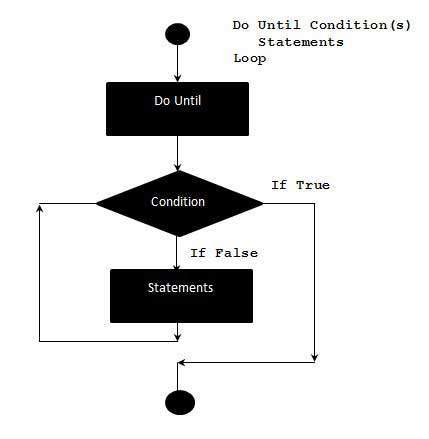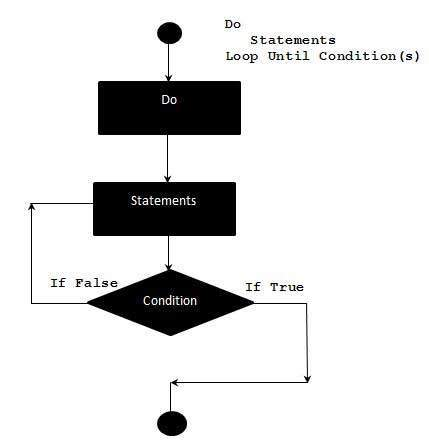# VBA - Do-Until Loops

A Do…Until loop is used when we want to repeat a set of statements as long as the condition is false. The condition may be checked at the beginning of the loop or at the end of loop.

## Syntax

Following is the syntax of a Do..Until loop in VBA.

```Do Until condition
[statement 1]
[statement 2]
...
[statement n]
[Exit Do]
[statement 1]
[statement 2]
...
[statement n]
Loop
```

## Flow Diagram### Example

The following example uses Do…Until loop to check the condition at the beginning of the loop. The statements inside the loop are executed only if the condition is false. It exits out of the loop, when the condition becomes true.

```Private Sub Constant_demo_Click()
i = 10
Do Until i>15  'Condition is False.Hence loop will be executed
i = i + 1
msgbox ("The value of i is : " & i)
Loop
End Sub
```

When the above code is executed, it prints the following output in a message box.

```The value of i is : 11

The value of i is : 12

The value of i is : 13

The value of i is : 14

The value of i is : 15

The value of i is : 16
```

## Alternate Syntax

There is also an alternate syntax for Do...Until loop which checks the condition at the end of the loop. The major difference between these two syntax is explained with the following example.

```Do
[statement 1]
[statement 2]
...
[statement n]
[Exit Do]
[statement 1]
[statement 2]
...
[statement n]
Loop Until condition
```

## Flow Diagram### Example

The following example uses Do...Until loop to check the condition at the end of the loop. The statements inside the loop are executed at least once, even if the condition is True.

```Private Sub Constant_demo_Click()
i = 10
Do
i = i + 1
msgbox "The value of i is : " & i
Loop Until i<15 'Condition is True.Hence loop is executed once.
End Sub
```

When the above code is executed, it prints the following output in a message box.

```The value of i is : 11
```
vba_loops.htm